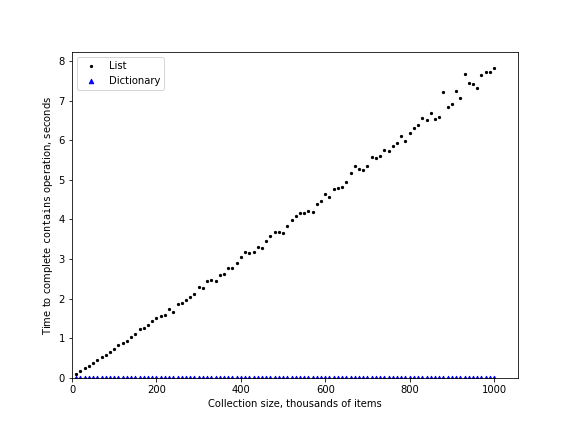# 2.7. Dictionaries¶

The second major Python data structure is the dictionary. As you probably recall, dictionaries differ from lists in that you can access items in a dictionary by a key rather than a position. Later in this book you will see that there are many ways to implement a dictionary. The thing that is most important to notice right now is that the get item and set item operations on a dictionary are $$O(1)$$. Another important dictionary operation is the contains operation. Checking to see whether a key is in the dictionary or not is also $$O(1)$$. The efficiency of all dictionary operations is summarized in Table 3. One important side note on dictionary performance is that the efficiencies we provide in the table are for average performance. In some rare cases the contains, get item, and set item operations can degenerate into $$O(n)$$ performance, but we will get into that in Chapter 8 when we talk about the different ways that a dictionary could be implemented.

Table 3: Big O Efficiency of Python Dictionary Operations

Operation

Big O Efficiency

copy

O(n)

get item

O(1)

set item

O(1)

delete item

O(1)

contains (in)

O(1)

iteration

O(n)

For our last performance experiment we will compare the performance of the contains operation between lists and dictionaries. In the process we will confirm that the contains operator for lists is $$O(n)$$ and the contains operator for dictionaries is $$O(1)$$. The experiment we will use to compare the two is simple: we’ll make a list with a range of numbers in it, then we will pick numbers at random and check to see if the numbers are in the list. If our performance tables are correct, the bigger the list, the longer it should take to determine if any one number is contained in the list.

We will repeat the same experiment for a dictionary that contains numbers as the keys. In this experiment we should see that determining whether or not a number is in the dictionary is not only much faster, but the time it takes to check should remain constant even as the dictionary grows larger.

Listing 6 implements this comparison. Notice that we are performing exactly the same operation, number in container. The difference is that on line 8 x is a list, and on line 10 x is a dictionary.

Listing 6

 1import timeit
2import random
3
4print(f"{'n':10s}{'list':>10s}{'dict':>10s}")
5for i in range(10_000, 1_000_001, 20_000):
6    t = timeit.Timer(f"random.randrange({i}) in x",
7    "from __main__ import random, x")
8    x = list(range(i))
9    lst_time = t.timeit(number=1000)
10    x = {j: None for j in range(i)}
11    dict_time = t.timeit(number=1000)
12    print(f"{i:<10,}{lst_time:>10.3f}{dict_time:>10.3f}")


Figure 4 summarizes the results of running Listing 6. You can see that the dictionary is consistently faster. For the smallest list size of 10,000 elements a dictionary is 89.4 times faster than a list. For the largest list size of 990,000 elements the dictionary is 11,603 times faster! You can also see that the time it takes for the contains operator on the list grows linearly with the size of the list. This verifies the assertion that the contains operator on a list is $$O(n)$$. It can also be seen that the time for the contains operator on a dictionary is constant even as the dictionary size grows. In fact, for a dictionary size of 10,000 the contains operation took 0.004 milliseconds, and for the dictionary size of 990,000 it also took 0.004 milliseconds.Figure 4: Comparing the in Operator for Python Lists and Dictionaries¶

Since Python is an evolving language, there are always changes going on behind the scenes. The latest information on the performance of Python data structures can be found on the Python website. As of this writing the Python wiki has a nice time complexity page that can be found at the Time Complexity Wiki.

Self Check